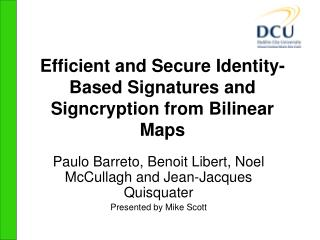Download PresentationEfficient and Secure Identity-Based Signatures and Signcryption from Bilinear Maps

# Efficient and Secure Identity-Based Signatures and Signcryption from Bilinear Maps - PowerPoint PPT PresentationDownload Presentation## Efficient and Secure Identity-Based Signatures and Signcryption from Bilinear Maps

- - - - - - - - - - - - - - - - - - - - - - - - - - - E N D - - - - - - - - - - - - - - - - - - - - - - - - - - -
##### Presentation Transcript

1. Efficient and Secure Identity-Based Signatures and Signcryption from Bilinear Maps Paulo Barreto, Benoit Libert, Noel McCullagh and Jean-Jacques Quisquater Presented by Mike Scott

2. A new Identity-Based Signature Scheme • Based on Sakai-Kasahara construction • Generators P, Q, g=e(P,Q), Master secret s, and Qpub=sQ, hash functions H1 and H2 • Keygen • D=1/(H1(ID)+s).P generates private key D from Identity ID and TA’s secret s

3. A new Identity-Based Signature Scheme • Signature • r=gx, for random x • h=H2(M,r), to sign a message M • S=(x+h)D, where D is the private key • Signature is (h,S)

4. A new Identity-Based Signature Scheme • Verification • Signature (h,S) is accepted iff • h = H2(M,e(S,H1(ID)Q+Qpub).g-h)

5. A new Identity-Based Signcryption Scheme • Parameters as before (with another hash function H3). Key generation as before. • Sign/Encrypt from Alice to Bob • r=gxfor random x, c=M  H3(r) • h=H2(M,r) • S=(x+h)DA, where DA is the senders private key • T=x(H1(IDB)P+Qpub), where IDB is recipient • Ciphertext is (c,S,T)

6. A new Identity-Based Signcryption Scheme • Decrypt/Verify • Compute r = e(T,DB), M=cH3(r), h=H2(M,r) • Accept iff • r = e(S,H1(IDA)Q+Qpub)g-h

7. Features • Fast and very efficient • No pairings for Sign/Encrypt • “Better than all the rest” – Tina Turner • Implemented and tested • Security proofs • Anonymity support